## Friday, September 5, 2014

### New function to ladderize tree with mapped discrete character

I just wrote a new utility function, `ladderize.simmap`, that can be used to “ladderize” a phylogenetic tree with a mapped discrete character. This function is analogous to the function `ladderize` in the ape package - in fact, it uses `ladderize` internally.

All ladderization does is rotate internal nodes to achieve an effect whereby right (or left) daughter clades tend to be more species rich than left daughter clades. Since node rotation is arbitrary, this can be done without any change in the phylogenetic information contained by the tree. The visual effect of ladderization will be strongest when the tree is highly imbalanced.

So, for instance, here is a ladderization of a simple pure-birth tree:

``````library(phytools)
packageVersion("phytools")
``````
``````##  '0.4.33'
``````
``````set.seed(890)
tree<-pbtree(n=200,scale=1)
plotTree(tree,ftype="off")
``````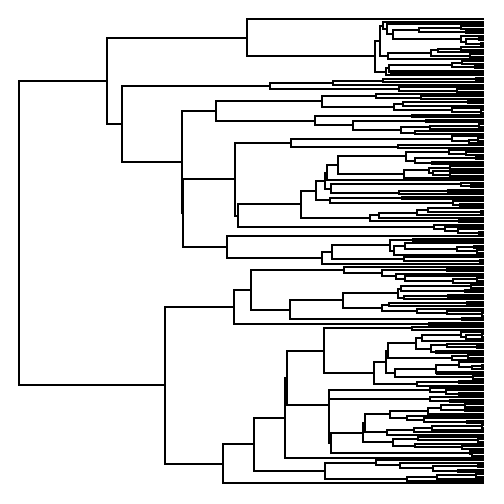``````plotTree(ladderize(tree),ftype="off")
``````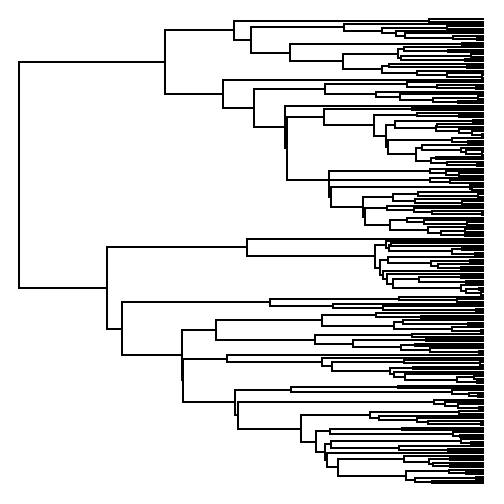Now, using a new version of phytools (phytools_0.4-33) let's try ladderizing an object with a mapped discrete character. In this case, we'll just create it using `sim.history` to simulate a discrete character history on the tree:

``````Q<-matrix(c(-2,1,1,1,-2,1,1,1,-2),3,3)
rownames(Q)<-colnames(Q)<-letters[1:3]
mtree<-sim.history(tree,Q)
plotSimmap(mtree,ftype="off")
``````
``````## no colors provided. using the following legend:
##        a        b        c
##  "black"    "red" "green3"
``````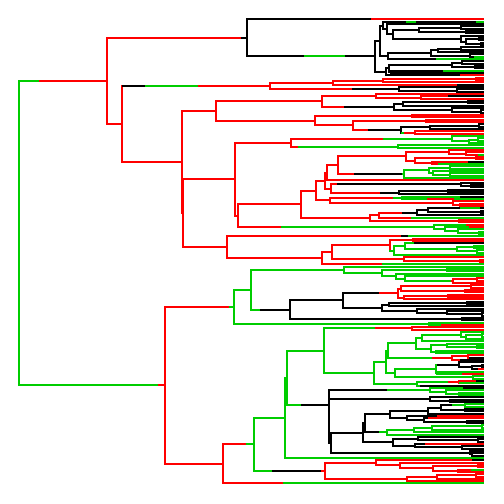``````plotSimmap(ladderize.simmap(mtree),ftype="off")
``````
``````## no colors provided. using the following legend:
##        a        b        c
##  "black"    "red" "green3"
``````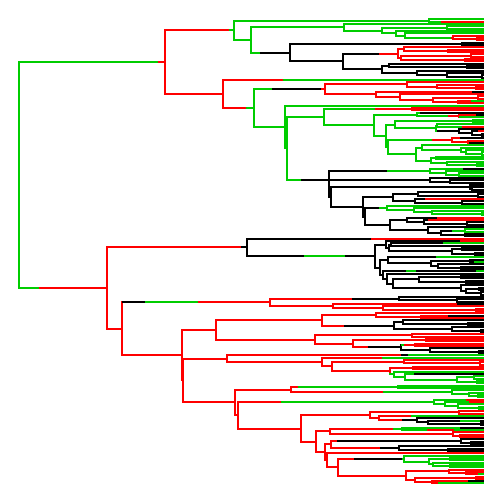Something else that's kind of cool is that we can also use the same method to ladderize objects of class `"densityMap"` and `"contMap"` since the tree is stored in the same way internally.

So, for instance:

``````set.seed(1)
x<-fastBM(tree)
obj<-contMap(tree,x,plot=FALSE)
plot(obj,ftype="off",lwd=3,outline=FALSE)
``````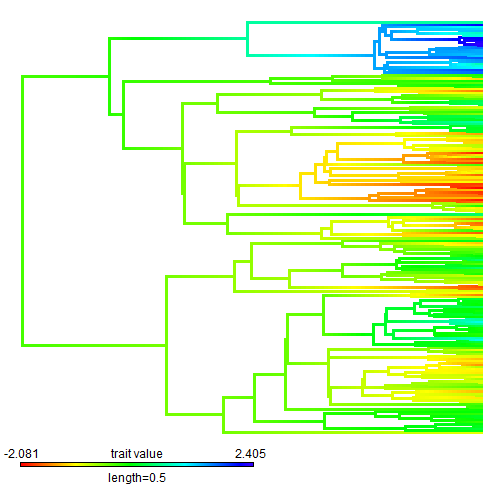``````obj\$tree<-ladderize.simmap(obj\$tree)
plot(obj,ftype="off",lwd=3,outline=FALSE)
``````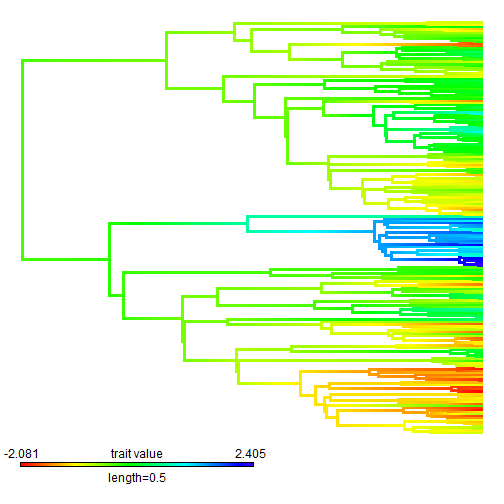This could be especially handy if our tree was crowding the color legend in the bottom left corner. (Of course, we can also move that, but this is easier).

That's it!

1.1.